## Exploring Heat and Temperature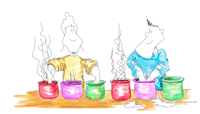In this unit students learn how to measure temperature in an objective way.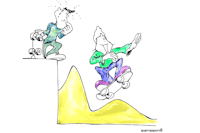In this unit students learn how to make and interpret temperature graphs.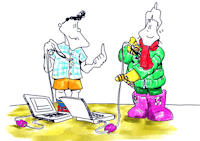In this unit students learn how to warm things up and discover the differences between heat sources and heat insulators.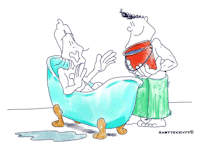In this unit students mix cold and hot water and make predictions and measurements of the resulting temperature of the mixtures.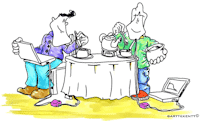In this unit students record the temperature during a process of cooling.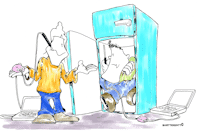In this unit students investigate in which ways the heat can be transferred and how to cool things faster.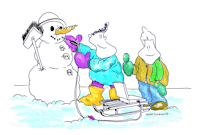In this unit students are introduced to the concept of melting. They find the melting temperature of ice and observe the change of this temperature after adding salt.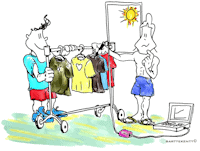In this unit students learn the concept of heat absorption.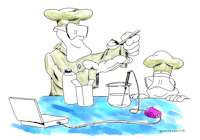In this unit students measure the temperature change during a chemical reaction of vinegar and baking soda.

## Exploring Light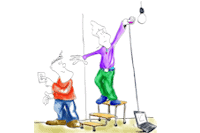In this unit students learn how to work with the €Sense's light sensor and measure light brightness of different light sources.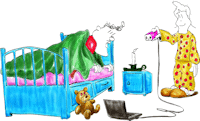In this unit students learn to make and interpret light intensity graphs.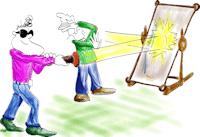In this unit students investigate how much light passes through different materials.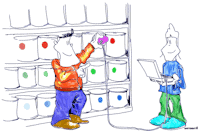In this unit students use the light sensor to measure light reflected from different materials.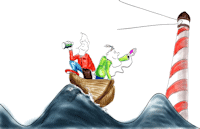In this unit student learn thet light is needed to see objects.

## Exploring Sound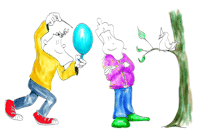In this unit students explore how sound is produced, they use the sound sensor to record the sound of a tuning fork and to display its sound waveform.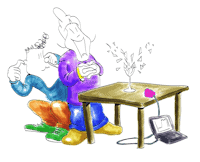In this unit students explore how sound is made by human voices.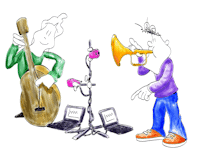In this unit students learn that sounds need a medium to travel and can travel not only via air but also via other materials.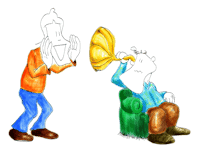In this unit students learn that sounds need a medium to travel and can travel not only via air but also via other materials.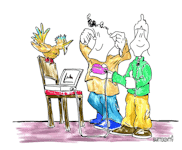In this unit students use the sound sensor and measure the sound intensity of different sounds. The sound sensor works as a sound level meter and measures sound level in decibels (dB).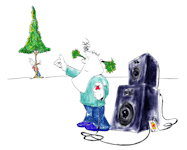In this unit students realise that sound can cause hearing damage and investigate ways of decreasing sound intensity.